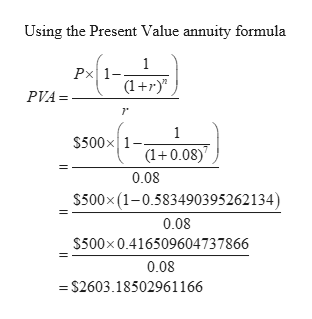# The present value of \$500 to be received at the end of each year for seven years at adiscount rate of 8 percent is ?

Question
1 views

The present value of \$500 to be received at the end of each year for seven years at a
discount rate of 8 percent is ?

check_circle

Step 1

Annuities are a regular stream of payments made for a defined period. Resent value of these annuities can b determined using a formula.

Step 2

Here,

Annuity payment ( P ) = \$500

Number of years ( n ) = 7

Discount rate (...help_outlineImage TranscriptioncloseUsing the Present Value annuity formula Px 1-. (1+r)" PVA = 1 \$500x|1- (1+0.08)' 0.08 \$500x (1-0.583490395262134) 0.08 \$500x0.416509604737866 0.08 = \$2603.18502961166 || fullscreen

### Want to see the full answer?

See Solution

#### Want to see this answer and more?

Solutions are written by subject experts who are available 24/7. Questions are typically answered within 1 hour.*

See Solution
*Response times may vary by subject and question.
Tagged in